omG8HI9LSKFW6lhCj8prTs0Z6lUhdOT9Jhi1Sf4m

# Three-Phase Electric Circuits: Balanced Wye-Wye Connection

The voltages we get from the three-phase power system are produced by a synchronous generator. In a balanced condition, the three voltages have equal amplitudes. Even they have equal amplitudes, which makes a three-phase system has three-phase voltages is their phase angle differences.

Each voltage has the 120◦ phase angle differences. It may be delta or wye, both have a difference of 120◦.

Make sure to read what is three-phase circuits first.

## Balanced Three-Phase Y Connection

We begin with the Y-Y system because any balanced three-phase system can be reduced to an equivalent Y-Y system. Therefore, the analysis of this system should be regarded as the key to solving all balanced three-phase systems.
A balanced Y-Y system is a three-phase system with a balanced Y-connected source and a balanced Y-connected load.
Consider the balanced four-wire Y-Y system of Figure.(1), where a Y-connected load is connected to a Y-connected source. We assume a balanced load so that load impedances are equal.

Although the impedance ZY is the total load impedance per phase, it may also be regarded as the sum of the source impedance ZS, line impedance Zl, and load impedance ZL for each phase, since these impedances are in series.

As illustrated in Figure.(1), ZS denotes the internal impedance of the phase winding of the generator; Zl is the impedance of the line joining a phase of the source with a phase of the load; ZL is the impedance of each phase of the load, and Zn is the impedance of the neutral line. Thus, in general(1)
ZS and Zl are often very small compared with ZL, so one can assume that ZY = ZL if no source or line impedance is given. In any event, by lumping the impedances together, the Y-Y system in Figure.(1) can be simplified to that shown in Figure.(2).Figure 1. A balanced Y-Y system, showing the source, line, and load impedances.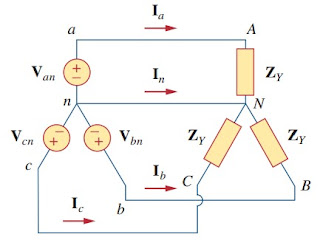Figure 2. Balanced Y-Y connection
Assuming the positive sequence, the phase voltages (or line-to-neutral voltages) are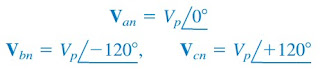(2)
The line-to-line voltages or simply line voltages VabVbc, and Vca are related to the phase voltages. For example,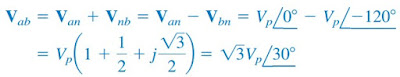(3a)
Similarly, we can obtain(3b)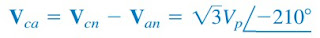(3c)
Thus, the magnitude of the line voltages VL is √3 times the magnitude of the phase voltages Vp, or(4)
where(5)
and(6)
Also, the line voltages lead their corresponding phase voltages by 30◦. Figure.(3a) illustrates this. Figure.(3a) also shows how to determine Vab from the phase voltages, while Figure.(3b) shows the same for the three-line voltages.

Notice that Vab leads Vbc by 120◦, and Vbc leads Vca by 120◦so that the line voltages sum up to zero as do the phase voltages.Figure 3. Phasor diagrams illustrating the relationship between line voltages and phase voltages
Applying KVL to each phase in Figure.(2), we obtain the line currents as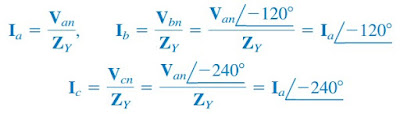(7)
We can readily infer that the line currents add up to zero,(8)
so that(9a)
or(9b)
that is, the voltage across the neutral wire is zero. The neutral line can thus be removed without affecting the system. In fact, in long-distance power transmission, conductors in multiples of three are used with the earth itself acting as the neutral conductor.

Power systems designed in this way are well grounded at all critical points to ensure safety.
While the line current is the current in each line, the phase current is the current in each phase of the source or load. In the Y-Y system, the line current is the same as the phase current. We will use single subscripts for line currents because it is natural and conventional to assume that line currents flow from the source to the load.Figure 4. A single-phase equivalent circuit
An alternative way of analyzing a balanced Y-Y system is to do so on a “per phase” basis. We look at one phase, say phase a, and analyze the single-phase equivalent circuit in Figure.(4). The single-phase analysis yields the line current Ia as(10)
From Ia, we use the phase sequence to obtain other line currents. Thus, as long as the system is balanced, we need only analyze one phase. We may do this even if the neutral line is absent, as in the three-wire system.

## Balanced Wye-Wye Connection Examples

For better understanding let us review the example below:
1. Calculate the line currents in the three-wire Y-Y system of Figure.(5).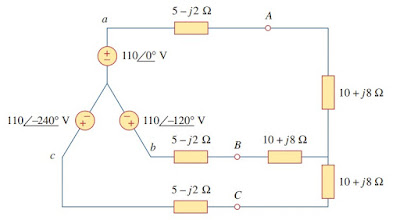Figure 5
Solution:
The three-phase circuit in Figure.(5) is balanced; we may replace it with its single-phase equivalent circuit such as in Figure.(4). We obtain Ia from the single-phase analysis as
where ZY = (5 − j2) + (10 + j8) = 15 + j6 = 16.155 21.8◦. Hence,
Since the source voltages in Figure.(5) are in positive sequence and the line currents are also in positive sequence,

Have you understood what is balanced wye-wye connection? Don't forget to share and subscribe! Happy learning!
Reference:  Fundamentals of electric circuits by Charles K. Alexander and Matthew N. O. Sadiku
Related Posts
SHARE